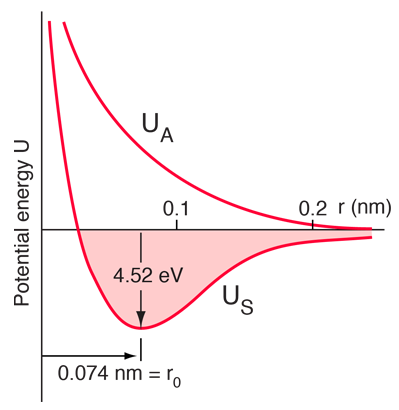Hydrogen Molecule

The classic case of covalent bonding, the hydrogen molecule forms by the overlap of the wavefunctions of the electrons of the respective hydrogen atoms in an interaction which is characterized as an exchange interaction. The character of this bond is entirely different from the ionic bond which forms with sodium chloride, NaCl. If you measure then energy balance when you form H+ and H- ions and examine the attractive force between them, the energy required is positive for any value of ion separation. That is, there is no distance at which there is a net attractive interaction, so the bond cannot be ionic.

The electron distribution around the protons of the hydrogen is described by a quantum mechanical wavefuntion, and the wavefunction which describes the two electrons for a pair of atoms can be symmetric or antisymmetric with respect to exchange of the identical electrons. From the Pauli exclusion principle, we know that the wavefunctions for two identical fermions must be antisymmetric. The electron spin part of the wavefunction can be symmetric (parallel spins) or antisymmetric (opposite spins), but then the space part of the wavefunction must be the opposite. That gaurantees that the entire wavefunction (the product of the spin and space wavefunctions) is antisymmetric. The two possibilities for the spatial wavefunctions for distant hydrogens are shown below.As shown below, when the hydrogen atoms are brought close together the symmetric spatial wavefunction leads to a bonding configuration of electrons and the antisymmetric one does not. The actual electron charge density is given by the square of the magnitude of the wavefunction, and it can be seen that the symmetric wavefunction gives a high electron density between the nuclei, leading to a net attractive force between the atoms (a bond).The exchange interaction (an entirely quantum mechanical effect) leads to a strong bond for the hydrogen molecule with dissociation energy 4.52 eV at a separation of 0.074 nm. The potential energy of the anti-bonding orbital shown gives some insight into why a third hydrogen atom cannot bond to the two atoms of the hydrogen molecule. It would be in an anti-bonding situation with one of the other hydrogen atoms and would therefore be repelled. We say that the bond in the hydrogen molecule is "saturated" because it cannot accept another bond.
 Chemical bonds
Index

Reference
Tipler
Elem. Modern Physics, Ch 5

 HyperPhysics***** Quantum Physics ***** Chemistry R Nave
Go Back